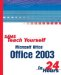# Recalculating Worksheets

 After you set up formulas, your job is done; Excel's job has just begun, however. If you change any value in the worksheet, Excel recalculates all formulas automatically! Therefore, Excel keeps your worksheet fresh and accurate as you modify values. You can use the same worksheet each month and change only the monthly data. If you leave the formulas intact, Excel computes and displays the correct answers. You can turn off the automatic recalculation and manually recalculate when ready if you use a slow computer and want to save time when editing a large worksheet. Select Tools, Options and click the Calculation tab. Click the Manual option to force manual recalculation. If you now change the data, Excel does not recalculate your worksheet until you press F9 (the Calculate Now shortcut key).Sams Teach Yourself Microsoft Office 2003 in 24 Hours
ISBN: 0672325535
EAN: 2147483647
Year: 2003
Pages: 272
Authors: Greg Perry